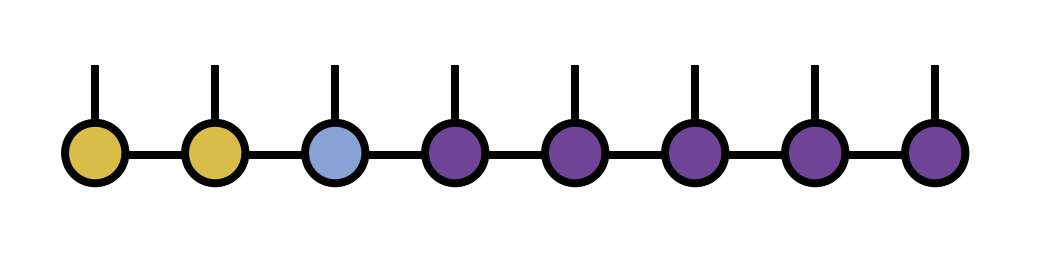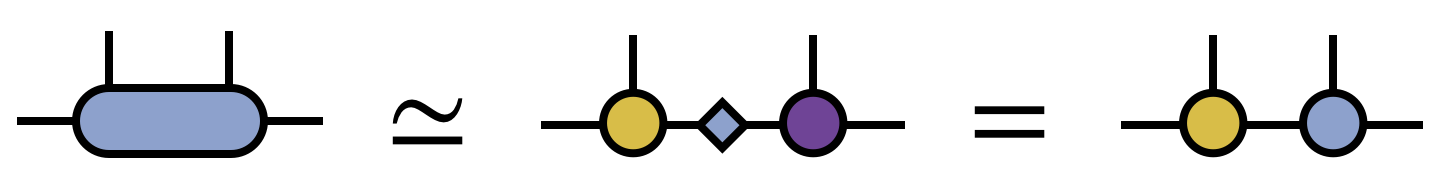# Applying a Two-site 'Gate' to an MPS

A very common operation with matrix product states (MPS) is multiplication by a two-site operator or 'gate' which modifies the MPS. This procedure can be carried out in an efficient, controlled way which is adaptive in the MPS bond dimension.

Say we have an operator $G^{s'_3 s'_4}_{s_3 s_4}$ which is our 'gate' and which acts on physical sites 3 and 4 of our MPS, as in the following diagram:To apply this gate in a controlled manner, first 'gauge' the MPS such that either site 3 or 4 is the 'orthogonality center'.

MPS psi; //psi is an object of type MPS
//... prepare psi, for example by initializing it to a particular state
psi.position(3); //shift the gauge position to site 3Next, contract the gate tensor G with the MPS tensors for sites 3 and 4auto wf = psi(3)*psi(4);
wf *= G;
wf.noPrime();


Finally, use the singular value decomposition (SVD) to factorize the resulting tensor, multiplying the singular values into either U or V. Assign these two tensors back into the MPS to update it.auto [U,S,V] = svd(wf,inds(psi(3)),{"Cutoff=",1E-8});
psi.set(3,U);
psi.set(4,S*V);


Note that in the SVD above, we set a truncation error cutoff to truncate the smallest singular values and control the size of the resulting MPS. Other cutoff values can be used, depending on the desired accuracy, as well as limits on the maximum bond dimension ("MaxDim").

Complete code example:

//assume we have an MPS 'psi' and a gate 'G'
//G is an ITensor with indices s3, s4, s3', s4'
//where s3 and s4 are the third and fourth physical
//indices of the MPS

psi.position(3);

auto wf = psi(3)*psi(4);
wf *= G;
wf.noPrime();

auto [U,S,V] = svd(wf,inds(psi(3)),{"Cutoff=",1E-8});
psi.set(3,U);
psi.set(4,S*V);Back to FormulasBack to Main